# Factors Divisibility Rules Exponents Prime Factorization and Greatest

• Slides: 13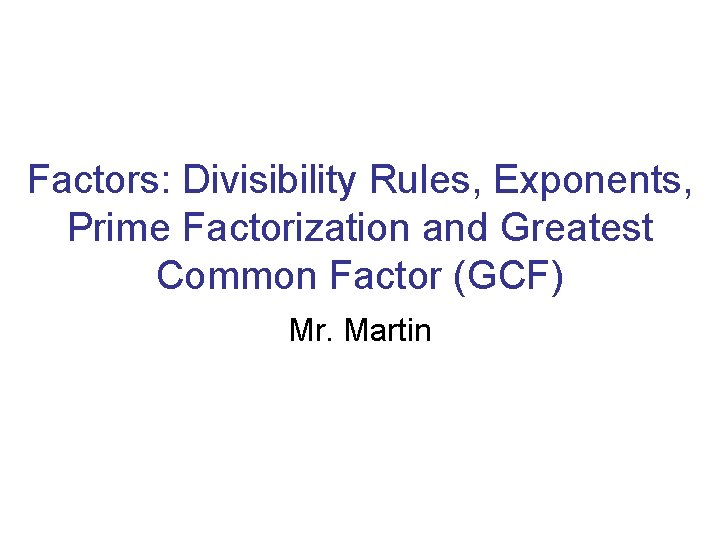Factors: Divisibility Rules, Exponents, Prime Factorization and Greatest Common Factor (GCF) Mr. Martin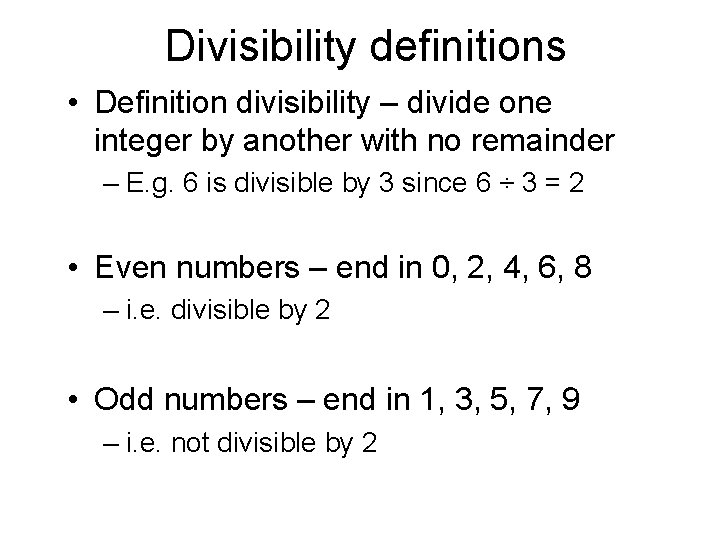Divisibility definitions • Definition divisibility – divide one integer by another with no remainder – E. g. 6 is divisible by 3 since 6 ÷ 3 = 2 • Even numbers – end in 0, 2, 4, 6, 8 – i. e. divisible by 2 • Odd numbers – end in 1, 3, 5, 7, 9 – i. e. not divisible by 2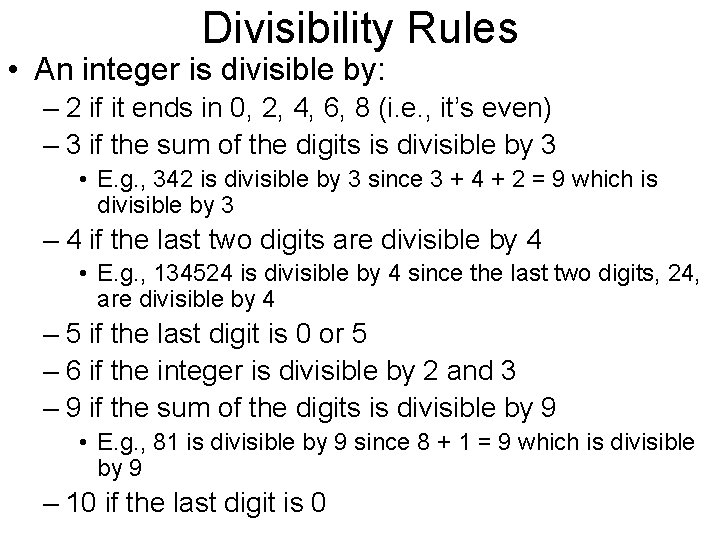Divisibility Rules • An integer is divisible by: – 2 if it ends in 0, 2, 4, 6, 8 (i. e. , it’s even) – 3 if the sum of the digits is divisible by 3 • E. g. , 342 is divisible by 3 since 3 + 4 + 2 = 9 which is divisible by 3 – 4 if the last two digits are divisible by 4 • E. g. , 134524 is divisible by 4 since the last two digits, 24, are divisible by 4 – 5 if the last digit is 0 or 5 – 6 if the integer is divisible by 2 and 3 – 9 if the sum of the digits is divisible by 9 • E. g. , 81 is divisible by 9 since 8 + 1 = 9 which is divisible by 9 – 10 if the last digit is 0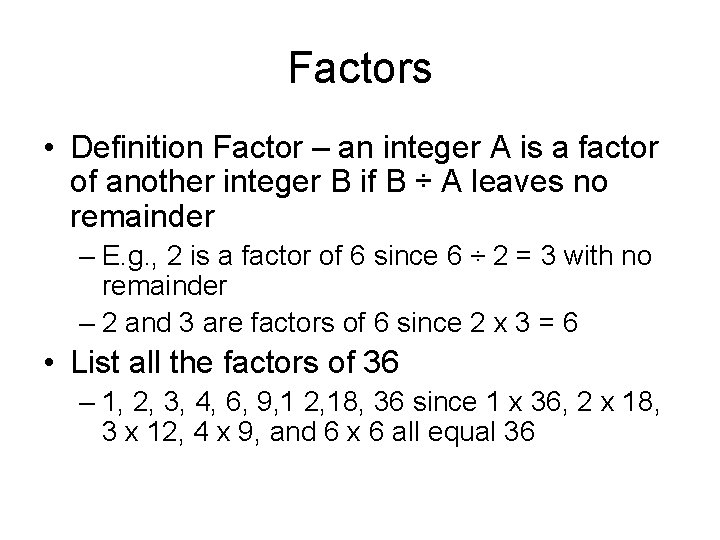Factors • Definition Factor – an integer A is a factor of another integer B if B ÷ A leaves no remainder – E. g. , 2 is a factor of 6 since 6 ÷ 2 = 3 with no remainder – 2 and 3 are factors of 6 since 2 x 3 = 6 • List all the factors of 36 – 1, 2, 3, 4, 6, 9, 1 2, 18, 36 since 1 x 36, 2 x 18, 3 x 12, 4 x 9, and 6 x 6 all equal 36Exponents • Exponents show repeated multiplication – E. g. , 43 = 4 x 4 = 64 • 4 is called the base and 3 is called the exponent • We read this “ 4 to the third power” or “ 4 to the power of 3” – E. g. , x 5 = (x)(x)(x) – E. g. , cm x cm = cm 3 • With numbers or variables to the second power, we often say “squared. ” For example, for 42 we can say “ 4 to the second power” or “ 4 squared. ” • With numbers or variables to the third power, we often say “cubed. ” For example, for 43 we can say “ 4 to the third power” or “ 4 cubed. ” • How do you think the terms “squared” and “cubed” came about? Think about area and volume.“Please excuse my dear Aunt Sally. ” • We can remember the proper order of operations by the sentence, “Please excuse my dear Aunt Sally, ” or “PEMDAS. ” • It stands for “Parenthesis, Exponents, Multiplication or Division (whichever occurs first), and Addition or Subtraction (whichever occurs first). • E. g. , Simplify 6(4 + 3)2. First, do the operation within the parenthesis. We get 6(7)2. Second, do the exponent. Since 7 x 7 = 49, we get 6(49). Now multiply 6(49) = 294. – BTW: I multiplied 6(49) in my head by using the distributive property. 6(50 – 1) = 6(50) – 6(1) = 300 – 6 = 294.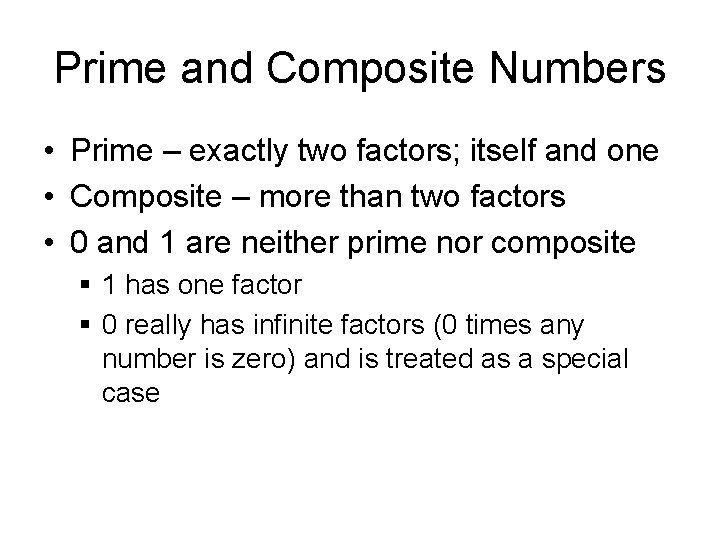Prime and Composite Numbers • Prime – exactly two factors; itself and one • Composite – more than two factors • 0 and 1 are neither prime nor composite § 1 has one factor § 0 really has infinite factors (0 times any number is zero) and is treated as a special casePrime Factorization • Prime factorization – expressing a number as the product of its prime factors – Usually done using a factor tree – Write final factors in increasing order from right to left – Use exponents for repeated factors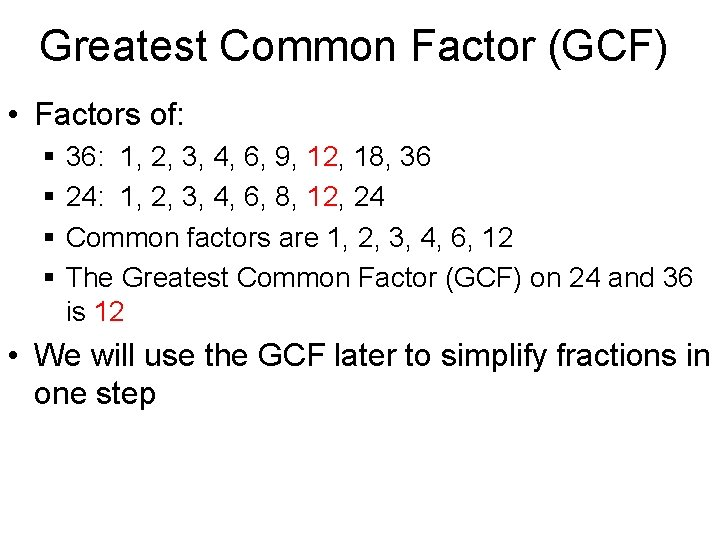Greatest Common Factor (GCF) • Factors of: § § 36: 1, 2, 3, 4, 6, 9, 12, 18, 36 24: 1, 2, 3, 4, 6, 8, 12, 24 Common factors are 1, 2, 3, 4, 6, 12 The Greatest Common Factor (GCF) on 24 and 36 is 12 • We will use the GCF later to simplify fractions in one step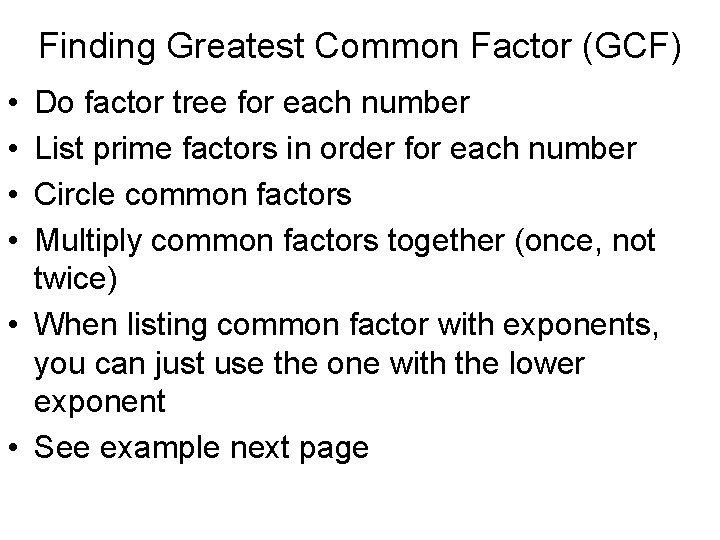Finding Greatest Common Factor (GCF) • • Do factor tree for each number List prime factors in order for each number Circle common factors Multiply common factors together (once, not twice) • When listing common factor with exponents, you can just use the one with the lower exponent • See example next page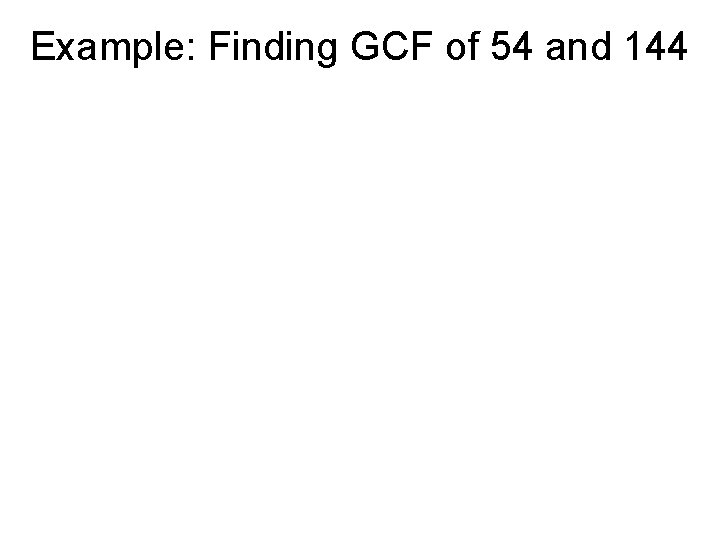Example: Finding GCF of 54 and 144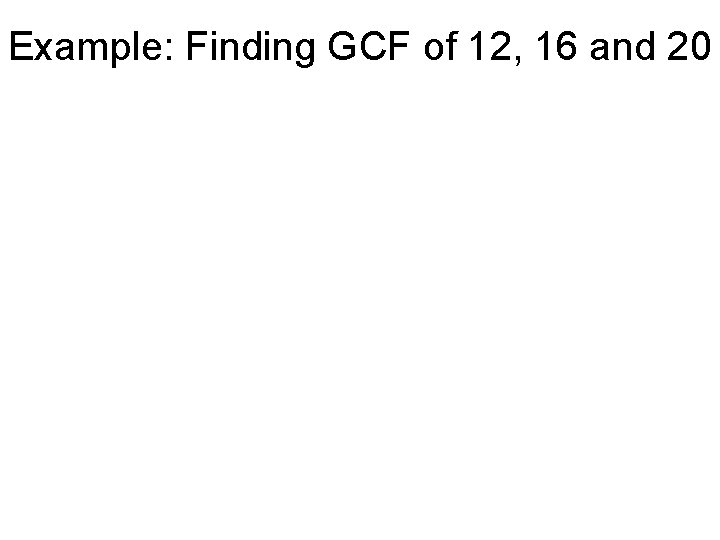Example: Finding GCF of 12, 16 and 20Ex: Finding GCF of 12 x 3 y and 18 x 2 y 2